## Abstract

The majority of the flowshop scheduling literature focuses on regular performance measures like makespan, flowtime etc.  In this paper a flowshop scheduling problem is addressed where the objective is to minimize completion time variance (CTV). CTV is a non-regular performance measure that is closely related to just-in-time philosophy.  A Microsoft Excel spreadsheet-based genetic algorithm (GA) is proposed to solve the problem.  The proposed GA methodology is domain-independent and general purpose.  The flowshop model is developed in the spreadsheet environment using the built-in formulae and function.  Addition of jobs and machines can be catered for without the change in the basic GA routine and minimal change to the spreadsheet model.  The proposed methodology offers an easy-to-handle framework whereby the practitioners can implement a heuristic-based optimization tool with the need for advanced programming tools.  The performance of the proposed methodology is compared to previous studies for benchmark problems taken from the literature.  Simulation experiments demonstrate that the proposed methodology solves the benchmark problems efficiently and effectively with a reasonable accuracy.  The solutions are comparable to previous studies both in terms of computational time and solution quality.

Keywords:  Completion time variance (CTV), flow shop, genetic algorithms, scheduling, spreadsheet

## 1. Introduction

Manufacturing plays a vital role in developing the economy as well as the human resources.  In today’s competitive world efficent use of materials and resources has become imperative to generate improved revenues.    Scheduling research primarily focuses on regular performance measures where the objective function increases as a function of job completion times.  Commonly used regular performance measures are: makespan, mean flowtime, tardiness, etc.  For regular performance measures, the determination of an optimal solution is relatively simple.  Most manufacturing compnaies nowadays prefer just-in-time (JIT) service or production systems.  Earliness and tardiness are both undesirable in a JIT environment.  CTV is one such non-regular perfomance measure that penalizes earliness as well as tardiness.  It is also closely related to quality of service provided to each customer.  CTV minimization is especially important when all jobs must spend the same time in the system, i.e., each job requires the same treatment.  Smaller CTV ensures more stable service.  CTV applications are found in many real-world applications such as production planning and internet data packet dispatching.

The first reported instance of the application of CTV minimization was proposed by Merten and Muller  in computer systems for solving the file organization problem where uniform response time was desired to be provided to users’ requests to retrieve data files.  Research has shown that an optimal CTV solution has a $V$-shape property . In a $V$-shaped optimal solution, the jobs before the smallest job are scheduled in decreasing order of the processing times, while the jobs after the smallest job are scheduled in an ascending order of the processing times.

Since the first application of CTV by Merten and Muller , most of the scheduling literature addressed CTV minimization in a single machine environment, e.g., Nasini and Nessah , Wang and Lu , Viswanathkumar and Srinivasan , Sharma , Gupta et al. , etc.  Minimization of CTV is considered $NP$-hard even for a single machine case . However, very little work has been reported for CTV minimization in a flowshop environment.

Flowshop scheduling research has attracted wide attention among researchers due to its many industrial as well as economic applications.  Some of the recent reviews highlighting the importance of flowshop scheduling has been presented by Çolak and Keskin , Lee and Loong  and González-Neira et al. . In a regular flowshop, a set of $n$-jobs are required to be processed on $m$-groups of machines.  Each of the $n$ jobs follows the same process sequence.  Minimization of CTV in a flowshop ensures uniform turnover of jobs and delivery to customer.

## 2. Literature review

The first instance of CTV minimization was reported by Marangos et al. . They applied a simulated annealing algorithm (SA) to minimize CTV in a two-machine flowshop.  The authors reported that the proposed approach obtains a solution in the range of 5-10% of the lower bounds.  Additionally, the SA algorithm can guarantee an optimal solution for problems having up to 10 jobs.  Gowrishankar et al.  addressed CTV minimization in an ${\textstyle n}$-jobs ${\textstyle m}$-machine flowshop.  The authors state that this is the first attempt to minimize CTV in an ${\textstyle n}$-jobs ${\textstyle m}$-machine flowshop.  A breadth first branch & bound procedure as developed to solve the problem.  Experimental analysis showed that the proposed method can effectively solve flowshop problems with CTV objective function.

Chandrasekaran et al.  applied a particle swarm optimization (PSO) algotithm to minimize CTV. Benchmark flowshop problems proposed by Taillard  are used to check the performance and efficiency of the proposed PSO.  The authors reported that the results obtained are better when compared with the previously published results.  Gajpal and Rajendran  applied an ant colony optimization (ACO) algorithm to minimize CTV.  The authors developed a new ACO algorithm (NACO) for the problem.  The performance of the proposed NACO is compared with exisiting ACO algorithms.  Experimental analysis showed that, on average, the proposed ACO finds better results as compared to exisiting ACO algorithms.

Chandrasekaran et al.  proposed a PSO algorithm for multi-objective flowshop scheduling problems where the objective is simultaneous minimization of flowtime, makespan and CTV.  The performance of the proposed PSO is enhanced by a local search mechanism.  Experimental analysis showed that the proposed PSO yields more non-dominated solutions as compared to other approaches.  Chen et al.  considered CTV minimization in an unrestricted parallel machine scheduling problem.  A heuristic algorithm is proposed to solve the problem.  The proposed heuristic algorithm can generate almost optimal schedules for small-sized problems.  Ponnambalam et al.  proposed a discrete PSO to solve a multi-objective flowshop scheduling problem where the objective is simultaneous minimization of flowtime, makespan and CTV. Computational analysis showed that the proposed discrete PSO performs better in terms of quality of solution as compared to previous studies.  Srirangacharyulu and Srinivasan  proposed a GA and a heuristic algorithm to minimize CTV in multi and single machine systems.  Comparative analysis demonstrated that the propsed algorithms can provide better results as compared to the exisiting algorithms.

Li et al.  considerd minimization of CTV in an unrestricted parallel machine environment and proposed an efficient heuristic Wavy Assignment, Verified Schedule (WAVS). The proposed algorithm produces near optimal solutions for small sized problems while outperforming existing algorithms for large sized instances.  Rameshkumar et al.  proposed two PSO algorithms: an ACO-inspired PSO and a discrete PSO.  Extensive computational analysis was carried out to compare the performance of the two proposed algortithms with previous studies for Taillard  benchmark problems.  On average, the proposed algorithm provides good and promising solutions.  Krishnaraj et al.  proposed an adaptation of ACO to minimize CTV in ${\textstyle n}$-jobs ${\textstyle m}$-machines flowshop scheduling problem.  Although the proposed algorithm does not provide best schedules for Taillard  benchmark problems, it does provide good and promising solutions for most of the instances.  Mehta et al.  proposed a two-phase local search algorithm to minimise CTV in a permutation flowshop.  In the first phase, an initial $V$-shaped solution is constructed using a local search method and then improved it to obtain a locally optimal $V$-shaped solution.  In the second phase, any possible sequences are traversed that may improve the solution.

Eren  considerd minimization of CTV for a two-machine flowshop.  A non-linear programming (NLP) method is proposed to solve the problem.  The proposed algorithm can effectively solve problems up to 30 jobs.  Problems up to 500 jobs are solved using a heuristic method. The author demonstrated that the proposed algorithm is very practical in solving real-world problems more effectively than the NLP models.  Lee et al.  considered minimization of total weighted completion time (TWCT) for identical parallel machine scheduling.  A two-agent based solution method is used to solve the problem.  For fewer number of jobs, a branch & bound procedure is also developed.  Rajkanth et al.  considerd minimization of CTV for single and identical parallel machines. A heuristic method is proposed to solve the problem.  The proposed heuristic method produces results better than the exisiting heuristics. It can also be modified and adapted to handle other non-regular performance measures like flow time variance and mean squared deviation as well as solve non-identical parallel machine problems.

Krishnaraj et al.  proposed a simulated annealing (SA) algorithm to minimize CTV in ${\textstyle n}$-job ${\textstyle m}$-machine flowshop shceduling problem.  The proposed algorithm works in three phases.  In the first phase, the algorithm minimizes CTV of jobs without right shifting of completion time (RSCT) of jobs.  In phase two, the algorithm minimizes CTV of jobs with RSCT of jobs, except the last job. In phase three, sequences are converted to follow a ${\textstyle V}$-shaped property with respect to processing time of jobs on the last machine, followed by RSCT except that of the last job so that the makespan and the machine utilization in the shop floor remain the same.  Extensive computational analysis was carried out with the existing heuristic algorithms.  The proposed algorithm is able to find good results.  Krishnaraj and Thiagarajan  also proposed a two-phase SA algorithm to minimize CTV in a flowshop environment.  The performance of the proposed SA algorithm is tested on 90 benchmark flowshop problems from Taillard . The proposed algorithm performs well in minimizing CTV especially in medium- and large-sized problems.

In this paper, we address the minimization of CTV in a flowshop environment using a general-purpose domain-independent GA embedded in a Microsoft Excel spreadsheet environment.  The performance of the proposed methodology is compared with existing heuristics for a wide range of benchmark problems taken from the literature.  Comparison is made with single machine, paralel machine and flowshop environments.

## 3. Problem definition & formulation of permutation flowshop scheduling problem

In a permutation flowshop shceduling problem, where the objective is to minimize completion time variance, ${\textstyle n}$ jobs from a set of ${\textstyle j=\{1,2,3,\cdots ,n\}}$ are to be processed on ${\textstyle m}$ machines ${\textstyle k=\{1,2,3,\cdots ,n\}}$. Each job ${\textstyle j}$ has a set of operations ${\textstyle \{o_{j1}}$, ${\textstyle o_{j2}}$, ${\textstyle o_{j3},\cdots ,o_{jk}\}}$ that are required to be processed through ${\textstyle m}$ machines requiring ${\textstyle p_{jk}}$ processing time.

Other assumptions used in the modeling of flowshop in this research application are as folows:

1. The number of ${\textstyle n}$ jobs and ${\textstyle m}$ machines are known before the planning horizon, i.e., ${\textstyle t=0}$.
2. All ${\textstyle n}$ jobs follow the same routing through the machines.
3. There is an umlimited buffer between the machines.
4. A different machine is required for the processing of each of the ${\textstyle o_{jk}}$ operations and there is only one machine of its kind in the flowshop.
5. A particular machine can process only one job at any given time and similarly, every job can only be processed by only one machine.
6. All processing times ${\textstyle p_{jk}}$ are known and deterministic.
7. Setup times for any operation, if any, are included in the processing time ${\textstyle p_{jk}}$.
8. All machines are continuously available and there is no breakdown of machines.
9. Once started, an operation cannot be stopped, i.e., no pre-emption is allowed.

The problem then is to efficiently schedule the job to minimize the completion time variance (CTV).  CTV for ${\textstyle n}$ jobs in a schedule is given by:

 $CTV=\,{\frac {1}{n}}\sum _{i=1}^{n}{\left({C}_{i}-{\overline {C}}\right)}^{2}$

where ${\textstyle {C}_{i}=}$completion time of job ${\textstyle i}$

 ${\overline {C}}={\frac {1}{n}}\sum _{i=1}^{n}{C}_{i}$

${\textstyle n}$ = total number of jobs.

## 4.  Proposed methodology

In the proposed methodology, a proprietry genetic algorithm Evolver 4.8  is used for the optimization.  Evolver works as an add-in to the Microsoft Excel spreadsheet.  The flowshop model for minimization of CTV is developed within the spreadsheet using the buil-in functions and formulae.

Research shows that, in the past few decades, spreadsheets have been extensivly used in various fields of engineering. Spreadsheets are popular among the practitioners because they eliminate tedious and repetitive calculations.  Some of the recent spreadsheet applications in engineering are: project scheduling , process planning and scheduling , airport ground crew scheduling , supply chain management (Amaral and Kuettner  and Othman et al. .

Evolver has also been used by many researchers for optimization problems of various applications.  Some of the recent applications of using Evolver are: risk analysis of reservoir operation , choosing materials for eco-friendly buildings , energy efficient operation of submersible pumps , operational optimization of a heat pump system , optimization of repetitive scheduling , optimum crew selection for repetitive projects .

The use of Microsoft Excel and Evolver optimization software offers an easy-to-handle framework for the practitioner.  This framework allows practitioners to implement a heuristic-based optimization tool without the need for advanced progamming skills.  Furthermore, Microsoft Excel and Evolver offer a user-friendly interface and facilitates user specification of the optimization parameters without the need for any programming skills.

The schematic diagram of spreadsheet – GA component integration is shown in Figure 1.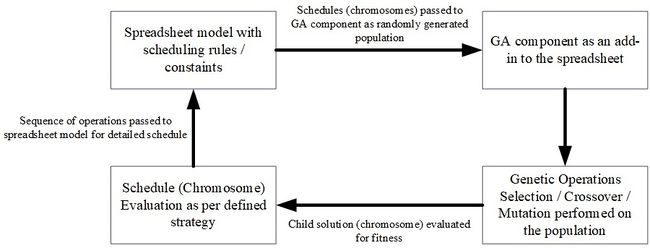Figure 1. Excel – GA component integration

Figures 2 and 3 show the pseudocode and flowchart respectively of the proposed algorithm.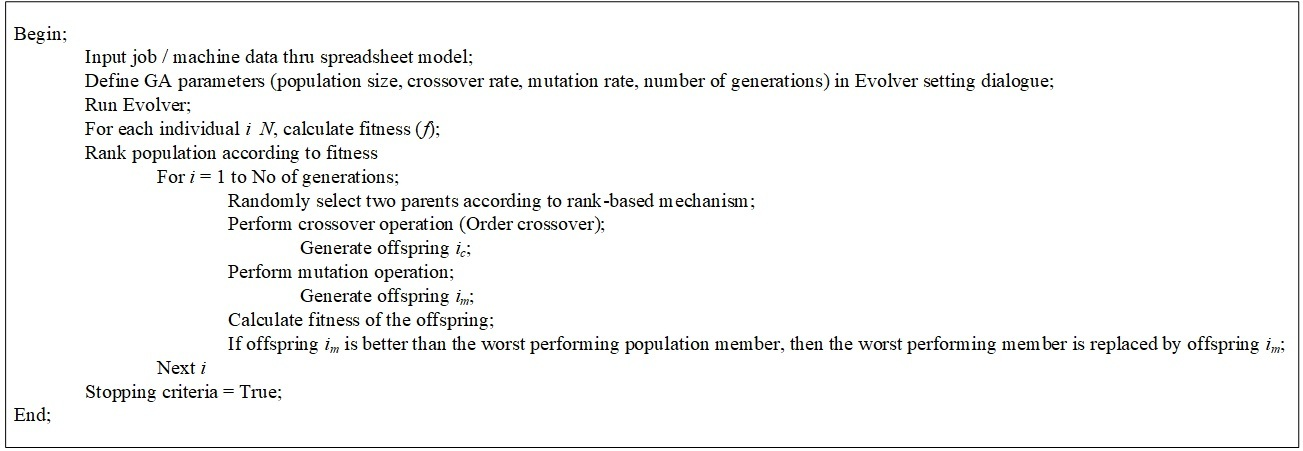Figure 2. Pseudocode of the proposed GA algorithm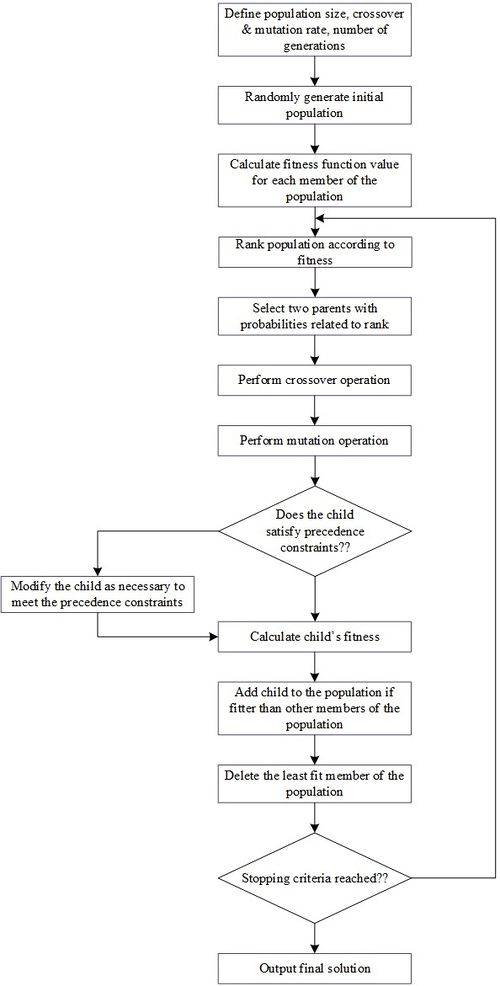Figure 3. Flowchart of the proposed GA algorithm

### 4.1 Chromosome representation

Permutation representation for chromosome (solution) is required to be used for the minimization of CTV in a flowshop environment. To address a particular problem situation, Evolver has various solving methods. The Order Solving method of Evolver is used to handle permutation representation. Permutation representation is described by considering six jobs D-C-A-B-E-F. In a permutation representation, the order of the genes are changed to formulate new solution strings. Chromosome F-C-B-A-E-D would be one of the possible chromosome representing permutation representation while another could be B-F-A-C-E-D.

### 4.2 Reproduction and selection

Steady state reproduction method  is used in Evolver. Unlike other reproduction techniques used in GA, steady state reproduction method produces only one child in each generation. The fitness of the child solution is compared to the fitness of other chromosomes in the population. In case the child solution is fitter, it replaces the worst performing member of the population, else it is discarded. For selection, Evolver uses rank-based selection method.

### 4.3 Crossover operator

Crossover operator combines genes from two parents to formulate new solutions. Order crossover  is used in the Order solving method. Order crossover randomy selects genes from one parent, then finds their place in the second parent. The remaining genes are copied in the second parent in the same order as they appear in the first parent. This ensures that the sub-ordering in the original parents are preserved while creating some new sub-orderings. Consider the two representing solutions in Figure 4.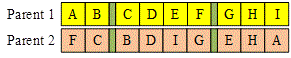Figure 4. Example parents for order crossover

The two cut points for each of the parents are indicated by green colour. The genes between the two cut points are copied in the child solution as they are in both parents, as shown in Figure 5.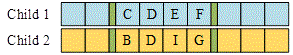Figure 5. Partial child solutions

Next, for child 1, starting from the second cut point of parent 1, the jobs from the second parent are copied, except those which are already in child 1, we get H-A-B- I-G. This sequence is placed in the first child. Similalry, the procedure is applied for child 2, where the genes are now copied from parent 1. The resulting child solutions would be as in Figure 6.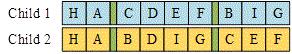Figure 6. Final child solutions

### 4.4 Mutation operator

With successive iterations, the population loses diversity and gets stuck in local optima. Mutation operator ensures that diversity is maintained in the population. The order solving method performs order-based mutation . In an order-based mutation, two positions are selected randomly, and then the genes in these position are swapped. For example, at position 3 and 6 in child 1 in Figure 6, we have genes C and F. By swapping these two genes, the resulting chromosome would be as shown in Figure 7.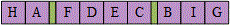Figure 7. Child solutions after mutation

## 5. Computation analysis

Simulation experiments were carried out to check the performance of the proposed methodology. Benchmark problems were taken from already published literature to validate the usefulness and performance of the proposed methodology. Evolver version 4.8 was used for the GA while Excel version 2003 was used to develop the flowshop models. The following GA parameters were used for the simulation experiments: crossover rate = 0.65, mutation rate = 0.07 and population size = 70. Each of the benchmark problems was run for 30 replications. The simulation experiments were conducted on a 2nd generation i7 laptop computer with 8GB RAM.

Comparison is made with various heuristics developed in previous studies based on relative percentage deviation (RPD) from the best found solution among all heuristics. The RPD is calculated as follows:

 $RPD=100\times {\frac {\left({CTV}_{proposed}-{CTV}_{best}\right)}{{CTV}_{best}}}\,$

where

${\textstyle {CTV}_{propsed}}$ = completion time variance result found by the proposed methodology, and

${\textstyle {CTV}_{best}}$ = best completion time variance found among all previous heuristics.

A positive RPD means that the CTV result found by the given heuristic is worse than the best CTV result among all heuristics, while a negative RPD means that the result found by a given heuristics is worse than the best CTV result.

### 5.1. Problem set 1

The first problem set is taken from Gupta et al. . The data set is a single machine scenario with jobs ranging from 10 to 20. The data set consists of 15 instances. Data for problem set 1 is presented in Table A1.

The perfomance of the proposed methodology is compared with results from GGA (GA by Gupta et al. ), HAS (hybrid simulated annealing algorithm by Mittenthal et al. ) and TSA (tabu search algorith by Al-Turki et al. ). The comparative analysis with three approaches is presented in Table 1.

Table 1. Comparative analysis of proposed methodology with previous studies for data set 1
Prob Instance No of Jobs Optimum Solution GGA HAS TSA Proposed GA
P1 10 7027.96 0 0 0 0
P2 10 12269.76 0 0 0 0
P3 10 20903.36 0 0 0 0
P4 10 14094.01 0 0 0 0
P5 10 18884.8 0 0 0 0
P6 15 16052.2 0.0044 0.0066 0 0
P7 15 27082.8 0.0029 0 0 0
P8 15 29805.4 0.0060 0 0 0
P9 15 57713.76 0.0025 0.0024 0 0
P10 15 36005.18 0.0006 0.0002 0 0
P11 20 70843.39 0.1806 0.0192 0 0
P12 20 76050.66 0.0333 0.0001 0.0006 0
P13 20 58912.59 0.0669 0 0 0
P14 20 54589.11 0.1972 0.0028 0.0001 0
P15 20 50015.15 0.1093 0.0016 0.0003 0
Average RPD 0.0402 0.0022 0.0001 0.0000

From Table 1, we can see that the average RPD for CGA, HAS, TSA and the proposed GA are 0.0402%, 0.0022%, 0.0001% and 0%, respectively. It is clear that, compared to CGA, HAS and TSA, the proposed GA methodology was able to find an optimal solution for all problem instances. The computation time required to find the optimum solution was less than 2 secs.

### 5.2. Problem set 2

Problem set 2 is taken from Srirangacharyulu and Srinivasan . Problem set 2 is also a sinlge machine scenario with jobs ranging from 15 to 25. The data set consists of nine instances. Data for problem set 2 is presented in Table A2.

The performance of the proposed methodology is compared with results from EC1.1 and EC1.2 (heuristic methods by Eilon and Chowdhury ), JJK (heuristic method by Kanet ), GGB (GA by Gupta et al. , MP (heuristic method by Prasad and Manna ), VS (verified spiral heuristic method by Ye et al. , HA (hybrid GA algorithm by Srirangacharyulu and Srinivasan ), SMH1 (heuristic algorithm by Rajkanth et al. ). The comparative analysis with three approaches is presented in Table 2.

Table 2. Comparative analysis of proposed methodology with previous studies for data set 2
Prob No of Jobs Optimum Result EC1.1 EC1.2 JJK MP GGB HA VS SMH1 Proposed GA
P1 15 38922.65 0.1669 0.1613 0.0003 0.0253 0.0003 0 0.0003 0.0012 0
P2 15 20102.38 0.2553 0.0882 0.0006 0.0049 0.0006 0 0.0006 0 0
P3 15 29217.09 0.0732 0.0061 0.0016 0.0016 0.0016 0 0.0016 0 0
P4 15 32551.32 0.1642 0.1351 0.0012 0.0072 0 0 0.0012 0 0
P5 20 64341.63 0.0898 0.0898 0.0030 0.0030 0.0009 0 0.0030 0 0
P6 20 51736.99 0.1830 0.1774 0.0049 0.0049 0.0474 0.0031 0.0049 0.0011 0
P7 25 107559.44 0.0550 0.0538 0.0003 0.0016 0.0002 0 0.0003 0.0003 0
P8 25 67358.88 0.0433 0.0434 0.0005 0.0006 0.0005 0 0.0005 0 0
P9 25 91018.42 0.0924 0.0686 0.0009 0.0013 0.0071 0.0006 0.0009 0.0015 0
Avg RPD 0.1248 0.0915 0.0015 0.0056 0.0065 0.0004 0.0015 0.0005 0

From Table 2, we can see that the average RPD for EC1.1, EC1.2, JJK, MP, GGB, HA, VS, SMH1 and proposed GA are 0.1248%, 0.0915%, 0.0015%, 0.0056%, 0.0065%, 0.0004%, 0.0015%, 0.0005% and 0%, respectively. It is evident from the results in Table 2 that the proposed GA was able to find an optimal solution for all problem instances of data set 2.

### 5.3. Problem set 3

Problem set 3 is taken from Li et al. . The problem set is an unrestricted parallel machine scernario and consists of nine jobs to be scheduled on two parallel machines to minimize CTV. The problem set consists of four subsets with processing times following four kinds of distributions: the uniform distribution of Uniform (1, 59), the normal distribution of Normal (30; 102), the exponential distribution of Exponential (30), and the Pareto distribution of Pareto (1.0345,1). Each of the subsets has five problem instances. The comparative analysis with WAVS algorithm  is given in Table 3 to Table 7.

The comparative results in Table 3 to Table 6 shows the superior performance of the proposed GA methodology with respect to WAVS heuritics. The proposed GA methodology was able to find an optimal solution in all the instances. In all the twenty instances, the best solution was obtained withn 4 secs on a 2nd generation i7 laptop computer with 8GB RAM.

Table 3. Comparative analysis of proposed methodology for data set 3 – Processing times from Uniform (1, 59)
Prob Instance Optimal CTV WAVS Proposed GA
CTV RPD CTV RPD
1 1512.85 1515.84 0.198 1512.85 0
2 831.28 840.4 1.097 831.28 0
3 322.74 324.98 0.694 322.74 0
4 737.5 737.5 0.000 737.50 0
5 630.59 632.53 0.308 630.59 0

Table 4. Comparative analysis of proposed methodology for data Set 3 – Processing times from Normal (30; 102)
Prob Instance Optimal CTV WAVS Proposed GA
CTV RPD CTV RPD
1 948.09 948.98 0.094 948.09 0
2 1719.99 1732.44 0.724 1719.99 0
3 849.74 858.49 1.030 849.74 0
4 1073.74 1074.59 0.079 1073.74 0
5 1525.98 1528.85 0.188 1525.98 0

Table 5. Comparative Analysis of Proposed Methodology for Data Set 3 – processing times from Exponential (30)
Prob Instance Optimal CTV WAVS Proposed GA
CTV RPD CTV RPD
1 333.9 335.23 0.398 333.90 0
2 1161.78 1162.74 0.083 1161.78 0
3 1102.99 1102.99 0.000 1102.99 0
4 2250.94 2255.94 0.222 2250.94 0
5 362.19 362.34 0.041 362.19 0

Table 6. Comparative analysis of proposed methodology for data set 3 – Processing times from Pareto (1.0345,1)
Prob Instance Optimal CTV WAVS Proposed GA
CTV RPD CTV RPD
1 225.5 316.38 40.302 225.50 0
2 70.19 70.19 0.000 70.19 0
3 78.99 78.99 0.000 78.99 0
4 137.84 139.09 0.907 137.84 0
5 41.13 41.19 0.146 1.13 0

### 5.4. Problem set 4

Problem set 4 consists of flowshop problems proposed by Taillard . The problem set has six different settings of $n$-jobs and $m$-machines. The data set consists of ${\textstyle 20\times 5}$, ${\textstyle 20\times 10}$, ${\textstyle 20\times 20}$, ${\textstyle 50\times 5}$, ${\textstyle 50\times 10}$, ${\textstyle 50\times 20}$ settings. Each setting has 10 problem instances thus, in total 60 different problems were solved to compare the performance of the proposed GA methodology with previously reported results. The problem instances can be accessed at http://mistic.heig-vd.ch/taillard/problemes.dir/ordonnancement.dir/ordonnancement.html.

The performance is compared with the following heuristics: SA – 2020 (simulated annealing heuristics by Krishnaraj and Thiagarajan , SA – 2019 (simulated annealing algorithm by Krishnaraj et al. ), ACO (ant colony algorithm by Gajpal and Rajendran ), MACO (modified ant colony algorithm by Krishnaraj et al. ), DPSO 1 (discrete particle swarm algorithm by Rameshkumar et al. ) and DPSO 2 (discrete particle swarm algorithm by Ponnambalam et al. . The comparative analyses for CTV results for all 60 instances are presented in Table 7 to Table 12.

It can be seen from Table 7 to Table 12, that the proposed methodology was able to find a solution very close to previously best known solutions. For ${\textstyle 20\times 5}$ problem instances, the proposed method provided the same solutions for five instances, and the average RPD was 0.03%. Overall, the proposed GA results were worse than SA – 2020 and DPSO 1.

For ${\textstyle 20\times 10}$ problem instances, the proposed method provided the same solutions for three instances with an average RPD of 0.23%. The proposed methodology solution was only better than ACO.

For ${\textstyle 20\times 20}$ problem instances, the proposed method provided the same solutions for two instances with an average RPD of 0.61%. In this case also, the proposed methodology solution was only better than ACO.

For ${\textstyle 50\times 5}$ problem instances, the proposed method did not find the same solutions for any of the instances. The average RPD was 0.61% for the proposed method. In this case too, the proposed methodology solution was only better than ACO.

Table 7. Comparative analysis of proposed methodology with previous studies for data set 4 – $20\times 5$
Instance SA - 2020 SA - 2019 ACO MACO DPSO 1 DPSO 2 Proposed GA
1 0.00 0.00 1.36 0.00 0.00 0.00 0.00
2 0.00 0.00 1.85 0.00 0.00 0.00 0.00
3 0.06 0.03 0.27 0.06 0.00 0.19 0.00
4 0.00 0.00 3.12 0.00 0.00 0.00 0.00
5 0.00 0.27 0.73 0.27 0.00 0.27 0.27
6 0.00 0.00 1.20 0.00 0.00 0.00 0.00
7 0.00 0.00 1.05 0.00 0.00 0.00 0.00
8 0.00 0.06 0.60 0.06 0.00 0.00 0.00
9 0.00 0.00 1.44 0.00 0.00 0.00 0.06
10 0.00 0.02 1.09 0.02 0.00 0.00 0.02
Avg RPD 0.01 0.04 1.27 0.04 0.00 0.05 0.03

Table 8. Comparative analysis of proposed methodology with previous studies for data set 4 – ${\textstyle 20\times 10}$
Instance SA - 2020 SA - 2019 ACO MACO DPSO 1 Proposed GA
1 0.00 0.00 2.38 0.00 0.00 0.45
2 0.00 0.67 0.85 0.85 0.00 0.67
3 0.00 0.00 1.28 0.00 0.00 0.00
4 0.00 0.00 0.00 0.00 0.00 0.22
5 0.00 0.07 1.49 0.07 0.00 0.00
6 0.00 0.00 0.00 0.00 0.00 0.06
7 0.00 0.00 7.97 0.00 0.00 0.00
8 0.87 0.87 2.10 0.87 0.00 0.87
9 0.00 0.31 0.61 0.31 0.00 0.00
10 0.00 0.00 4.73 0.00 0.00 0.00
Avg RPD 0.09 0.19 2.14 0.21 0.00 0.23

Table 9. Comparative analysis of proposed methodology with previous studies for data set 4 – ${\textstyle 20\times 20}$
Instance SA - 2020 SA - 2019 ACO MACO DPSO 1 Proposed GA
1 0.00 0.00 3.86 0.00 0.00 0.67
2 0.00 0.00 1.90 0.00 0.00 0.65
3 0.30 0.30 0.30 0.30 0.00 0.47
4 0.00 0.00 1.23 0.00 0.00 0.00
5 0.00 0.00 1.21 0.00 0.00 0.00
6 0.00 0.00 0.00 0.00 0.00 0.00
7 0.00 0.00 1.30 0.00 0.00 0.96
8 0.00 0.00 4.51 0.00 1.27 3.13
9 0.00 0.99 1.07 0.99 0.00 0.00
10 1.48 0.00 3.03 0.00 0.00 0.24
Avg RPD 0.18 0.13 1.84 0.13 0.13 0.61

Table 10. Comparative analysis of proposed methodology with previous studies for data set 4 – $50\times 5$
Instance SA - 2020 SA - 2019 ACO MACO DPSO 1 Proposed GA
1 0.12 0.00 0.99 0.43 0.25 0.91
2 0.00 0.02 1.54 0.02 0.37 0.39
3 0.28 0.46 2.51 0.46 0.00 1.24
4 0.00 0.29 1.46 0.66 0.29 0.84
5 0.00 0.10 1.21 0.10 0.05 0.22
6 0.04 0.00 0.76 0.22 0.03 0.33
7 0.00 0.40 0.88 0.54 0.42 0.19
8 0.00 0.10 1.13 0.10 0.10 0.44
9 0.09 0.00 1.08 0.42 0.06 0.74
10 0.02 0.00 1.06 0.07 0.00 0.25
Avg RPD 0.05 0.14 1.26 0.30 0.16 0.55

Table 11. Comparative analysis of proposed methodology with previous studies for data set 4 – $50\times 10$
Instance SA - 2020 SA - 2019 ACO MACO DPSO 1 Proposed GA
1 0.22 0.00 1.03 0.54 0.80 2.14
2 0.00 0.51 3.45 1.02 2.93 3.22
3 0.55 0.00 1.21 1.13 0.04 3.43
4 0.13 0.00 2.53 0.61 0.83 1.98
5 0.00 0.14 0.54 0.14 0.10 1.42
6 0.66 0.86 2.79 0.86 0.00 2.29
7 0.00 0.51 1.79 0.87 0.18 1.86
8 0.00 1.30 2.37 1.30 1.72 2.02
9 0.00 0.65 2.27 1.17 0.45 2.34
10 0.02 0.22 0.86 0.86 0.00 1.23
Avg RPD 0.16 0.42 1.88 0.85 0.70 2.19

Table 12. Comparative analysis of proposed methodology with previous studies for data set 4 – $50\times 20$
Instance SA - 2020 SA - 2019 ACO MACO DPSO 1 Proposed GA
1 0.66 0.00 1.82 0.46 0.35 3.25
2 1.24 0.00 2.33 0.00 1.17 3.82
3 0.03 0.47 0.47 0.47 0.00 3.25
4 0.00 0.63 1.57 1.12 1.15 4.57
5 0.00 0.76 5.03 3.07 0.90 2.77
6 0.00 0.45 0.80 0.65 0.80 3.43
7 0.55 0.00 2.51 1.26 0.37 2.99
8 0.91 0.00 5.60 2.43 1.75 6.12
9 0.00 0.77 2.79 1.81 2.79 4.65
10 0.29 1.30 1.61 1.61 0.00 6.82
Avg RPD 0.37 0.44 2.45 1.29 0.93 4.17

For $50\times 10$ problem instances, the proposed method did not find the same solutions for any of the instances. The average RPD was 2.19% for the proposed method. For $50\times 10$ problem instances, the proposed method did not find the same solutions for any of the instances. The average RPD was 4.17% for the proposed method.

From the discussion in the preceding paragraphs, we can see that the proposed GA methodolgy found optimal solutions fo all single machine and unrestricted parallel machine scenarios. For $n\times m$ flowshop problems, the solution quality deteriorated as the problem size increased. However, for small-to-medium-sized problems, the soultion quality of the proposed methodology was comparable with other heuristic techniques.

## 6. Conclusions

Most of the research on flowshop scheduling addresses the problem with regular performance measures like, makespan, flowtime etc. Very little work has been carried out with non-regular performance measures. Completion time variance (CTV) is one of the non-regular objective functions that penalizes both earliness and tardiness. CTV has direct relevance with JIT where it is desired that a job is completed exactly on time, neither early, as it will incur inventory holding cost, nor late, as it will result in products being delivered late thus, resulting in bad reputation for the company.

In this research, minimization of completion time variance (CTV) in a flowshop was addressed. A general purpose domain-independent GA Evolver that works as an add-in to Microsoft Excel spreadsheet was used for the optimization of the schedules. The shop models for flowshop were made in the spreadsheet using the built-in functions and formulae.

The proposed approach offers an easy-to-handle framework for the practitioners who are used to tools like spreadsheets. The proposed methodology allows practitioners to implement a heuristic-based optimization tool without the need for advanced progamming skills. Furthermore, due to the arrangement of data in linked cells via the spreadsheet, it is very convenient to carry out what-if analysis thus, looking at the effect of change due to various parameters. Additionally, the proposed approach can be used to optimize any objective function without the need to change the spreadsheet model of the basic GA routine. The proposed methodology can also be enhanced with the use of Viusal Basic for Application (VBA) language available within the Excel spreadsheet.

Even though the performance of the proposed methodology was not very promising for large-sized problems, computational experiments demonstrated that the proposed methodology can find optimal solutions for small-to-medium-sized problems.

## Acknowledgements

This research has been funded by Scientific Research Deanship at University of Ha’il – Saudi Arabia through Project number RG – 20 028.

### Document informationPublished on 02/06/22
Accepted on 22/05/22
Submitted on 28/02/22

Volume 38, Issue 2, 2022
DOI: 10.23967/j.rimni.2022.05.002

### Document Score0

Views 98
Recommendations 0